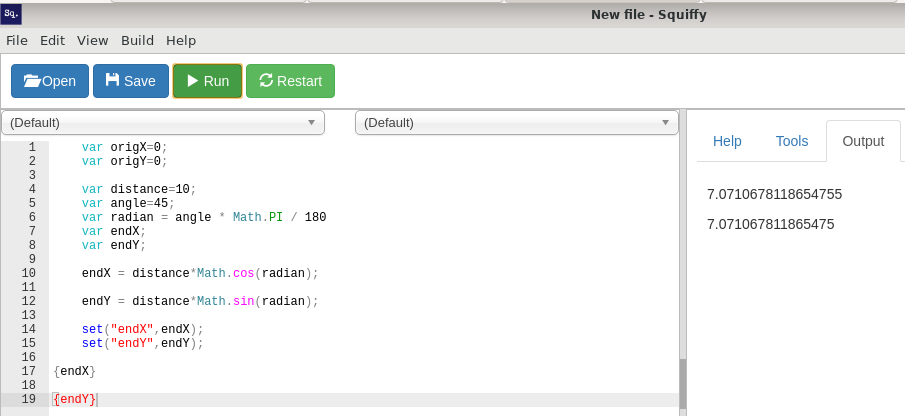## Anyone remember triginometry?Trying to get this to work. Basically, I need to know (if you are traveling along an angle) what your grid location is.

``````    var origX=0;
var origY=0;

var distance=10;
var angle=45;

var endX;
var endY;

endX = distance*Math.cos(angle);

endY = distance*Math.sin(angle);

set("endX",endX);
set("endY",endY);

{endX}
{endY}
``````

A 45 degree angle should ensure endX and endY are equal but they are not. All my other trig functions work but these do not. Ideas?Over my head, but this page might have a formula or two (accompanied by the JS scripts) which may help out a little.For some reason... Computers are set up to use radians instead of degrees...
(MAYBE 1 % of the people that would use trigonometry use radians.)
degrees = radians × 180° / π
radians=degrees x π/180°
So: your heading is 45° (angle)
R=angle * (Pi/180)
endX = distanceMath.cos(R);
endY = distance
Math.sin(R);For some reason... Computers are set up to use radians instead of degrees...

It's because trigonometry works in radians. Take this example:

``````function sine(angle) {
var result = angle;
var j = angle;
var k = 1;
for (i=0 ; i<10 ; i++) {
j *= -angle*angle;
k *= i * (i+1);
result += j/k;
}
return result;
}
``````

Calculating sin just using addition and multiplication. If your calculator has a 'degrees' mode, it will convert your angle into radians before using that function.

So, that's why computers use it. Because it's the natural

(MAYBE 1 % of the people that would use trigonometry use radians.)

I guess architects and surveyors maybe? Not sure. The only people I know who do trig stuff on a daily basis are programmers and mathematicians, and much more likely to use radians and steradians.So, I assume (after compiling information supplied by everyone) that Bluevoss wants this(?):

``````var origX=0;
var origY=0;

var distance=10;
var angle=45;
var radian = angle * Math.PI / 180
var endX;
var endY;

endX = distance*Math.cos(radian);

endY = distance*Math.sin(radian);

set("endX",endX);
set("endY",endY);

{endX}

{endY}
``````PS

DL!

Whassup!!!You know, I'd just got another test section working to find the angle - struggled with it until I found the bit about converting radians. I didn't know you needed to convert them back (which, as I write this, seems pretty damn stupid of me).

The problem is that most of the pages I look at give the formulas for determining the angles (which was easy enough) but fail to mention the conversion to and from Radians. Sheesh. Worst sites were the ones that came up when I searched "how to determine sides right triangle" (or something like that) and provided a calculator. I don't want a calculator - I want to know how to do it!

Anyway, thanks all who provided insight on this. I used to be pretty good in college physics and such, but that was 35 years ago.I didn't know you needed to convert them back

You probably don't.
Two main methods:

1. Store your angles in degrees, and convert them to radians whenever you want to do maths with them.
2. Store radians, and convert them to degrees when you want to display a bearing to the player (or convert to radians if you're asking the player for a heading).

Which method is better probably depends how often you do each thing. How often do you find the sin or cos of an angle; vs how often do you need to display a number of degrees to the player?Um... never need to display.

I think I'd like to talk to my lawyer, now.

This topic is now closed. Topics are closed after 60 days of inactivity.

Quest
Squiffy
ActiveLit

Documentation
Blog
GitHub

Forums